# How Are Exercise Balls Measured?

Gone are the days when an exercise ball only meant one size. Today, exercise balls range from large to small, depending on their use.

This post talks about the popular large exercise balls you see in yoga studios, gyms, and therapy rooms. These large exercise balls are commonly used to improve core strength and balance.

If you are looking for an exercise ball to use at home, you are probably wondering what size of ball you should buy. And if you don’t know the size just yet, that’s understandable. It can be confusing for a lot of us who are new to this exercise equipment.

First, you must know how you will use an exercise ball. That way, you will know what ball size you need.

If you determine you need a large exercise ball, know there’s a standard to how these balls are measured.

This article will prepare you for your next visit to a sports equipment store. It will tell you everything you need to know, including the kind and size of ball you’ll need for your exercises.

## How Are Exercise Balls Measured?The first thing you have to know is how exercise balls  are measured. Knowing this will help you find the right exercise ball for your height.

As mentioned earlier, there is a standard to measuring an exercise ball. Exercise balls are measured by getting the diameter of the ball and denoting it in centimeters.

The diameter of a ball is the straight length across one side of a ball. It is the distance between the leftmost part of the ball to the rightmost part of the ball.

If you want to measure the circumference of the ball, place the tape measure around the widest part of the ball. You can wrap it from side to side or from top to bottom, and still get the same measurements.

A large exercise ball is between 55 to 75 centimeters in diameter. These large exercise balls are broken down into three sizes: 55, 65, and 75 centimeters.

Exercise balls  used in physical therapy are much smaller. They are about the size of your palm. This is mainly because they’re used for pressure points and myofascial release.

Some exercise balls label the diameter in millimeters. This applies to smaller exercise balls used in therapy or for arm toning exercises.

If you see an exercise ball whose diameter is denoted in inches, you can convert it to centimeters by multiplying the length by 2.54.

## How Do You Measure an Exercise Ball?

There are several ways to measure an exercise ball. One uses a tape measure and the other uses a wall and box method.

### The Tape Measure Method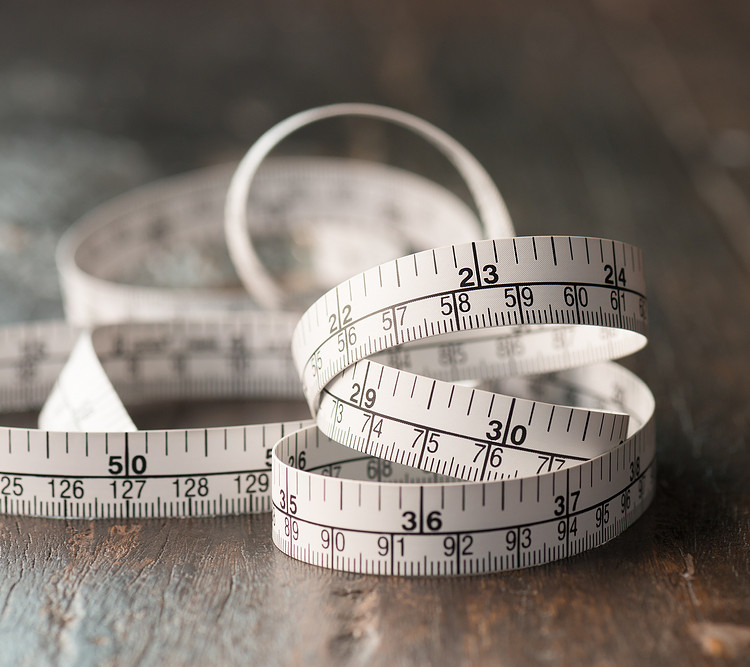The easiest method is to use a tape measure. Here are the steps to do it:

1. Get a fully inflated exercise ball.
2. Wrap the tape measure around the widest area of the ball to know the circumference. This is the midsection of the ball.
3. Record the circumference of the ball in centimeters. Then, divide the number using pi or 3.14.

The answer to this formula is the diameter of your exercise ball. If you are getting the radius of your exercise ball, just divide the diameter by two.

### The Wall and Box Method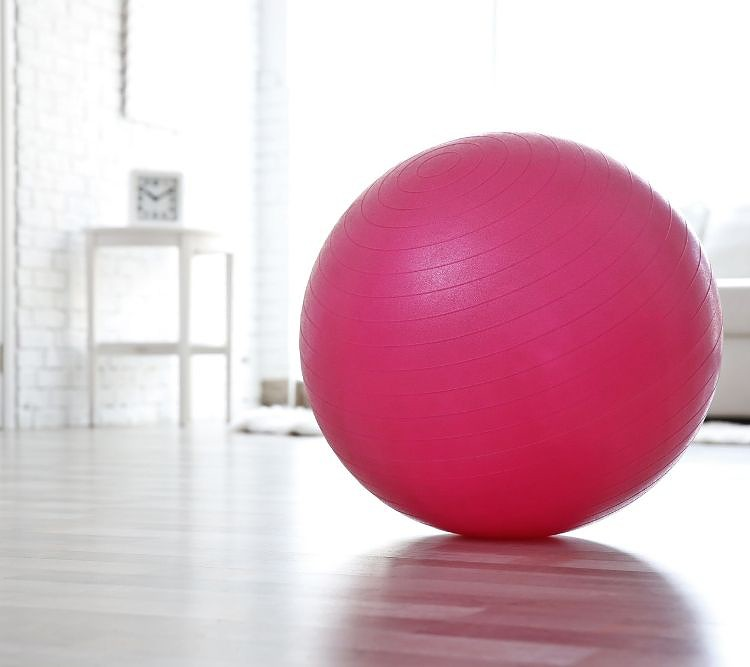The other method is to use a wall and a box. Here are the steps to measure the exercise ball using this method:

1. Put the exercise ball beside a wall. Make sure that the side of the ball touches the wall.
2. Get a box, ideally a large box that has some weight to it. It should not be lighter than the ball because the ball won’t be able to stay in place.
3. Place a fully inflated exercise ball between the wall and the box. The ball should not be squished between the wall and the box. There should not be any added pressure to the sides of the ball.
4. Measure the distance between the wall and the box. Make sure the measuring tape is straight to get accurate measurements.

If you’re getting the radius, just divide the diameter in two.

## What Size of Exercise Ball Should I Sit on?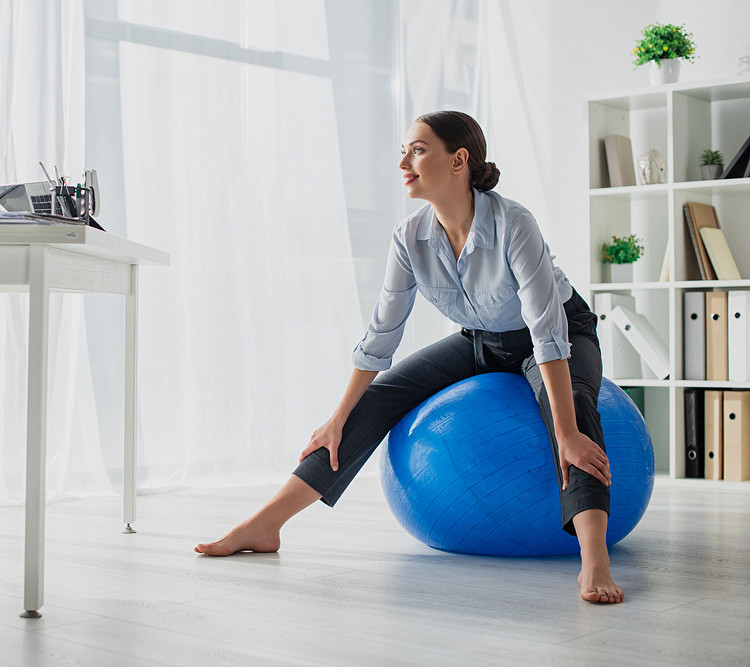There are two ways to determine the size or height of an exercise ball  ideal for your height.

The first method is by using your body. All you have to do is sit on an inflated exercise ball and ask yourself these questions:

• Can I sit in an upright position?
• Are my thighs parallel to the floor?
• Do my feet rest flat on the ground?
• Do my legs form a 90-degree angle?

If the answer is yes for all of these things, you have found the right exercise ball size for you.

On the other hand, if you sit on a ball and you find that your shins have to be in a slanted position, the exercise ball is too small or it needs more air.

While sitting on the ball, if your feet cannot touch the ground, the ball is too big for you. Buying a larger exercise ball than what your height needs will make the ball unstable and hard to use for your exercises.

When measuring an exercise ball using your body, always make sure the ball you’re “testing” is properly inflated. If it isn’t, you will struggle with identifying the right size for your height.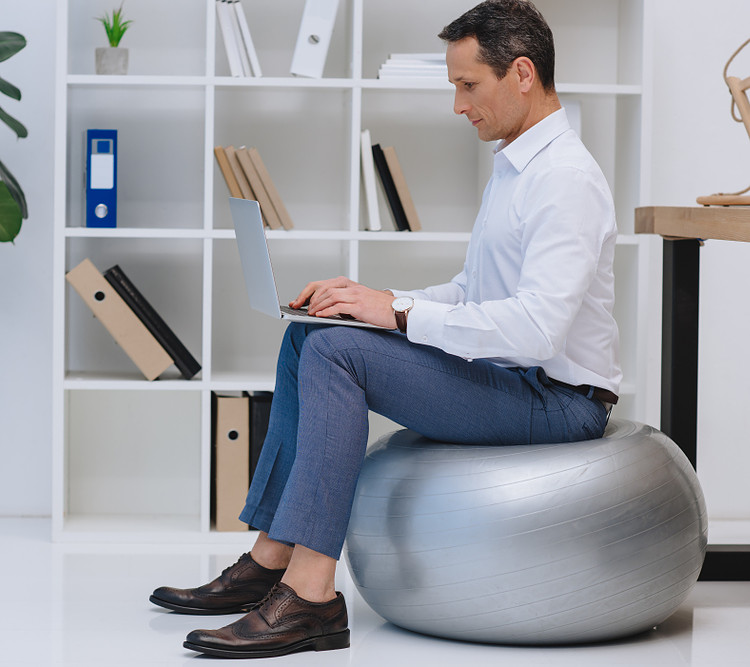Since exercise balls  are used for sitting, standing, and stretching, we recommend you sit on an exercise ball to determine if you are comfortable with its height.

The second method of measuring the correct ball size for your height is using a guide for the right exercise ball size. If your height is between 155 to 170 centimeters (5’1” to 5’7”), the ideal ball size for you is a 55-centimeter exercise ball.

For those of you who are 173 to 185 centimeters tall (5’8” to 6’1”), choose a 65-centimeter ball. If you are taller than 188 centimeters (6’2”), you should use a 75-centimeter ball.

These measurements apply to large exercise balls only. Different types and sizes of exercise balls will have other size charts based on your height or weight.

### Knowing if You Have the Right Exercise Ball Size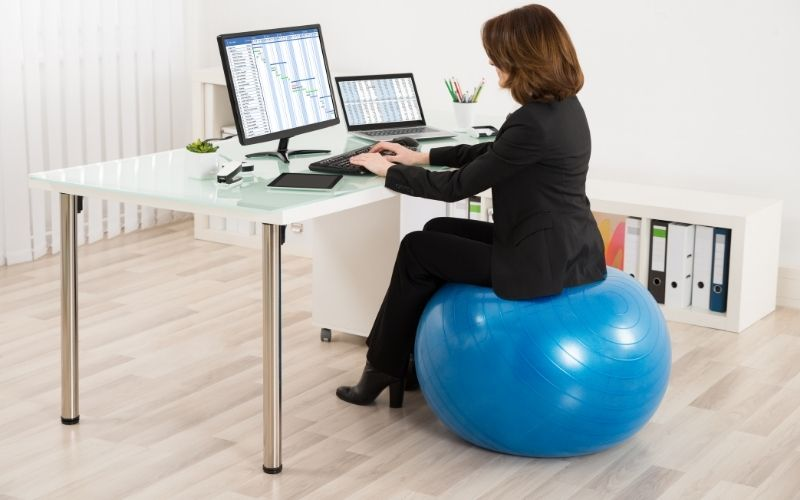There is another way to know if you have the right exercise ball apart from sitting on it.

When you sit on a fully inflated exercise ball , the difference in the height of the ball before you sit on it to after you sit on it should not be more or less than six inches.

You can measure the difference by first measuring the height of the ball before you sit on it. Second, you will measure the height of the ball while you are sitting on it.

Subtract the first measurement from the second and the difference should be six inches.

If the difference is more than six inches, it means your exercise ball needs to be inflated with more air. Fill your ball with air until the exercise ball falls within the six-inch range. Make sure you don’t overfill your ball.

## Different Sizes of Exercise Balls According to Type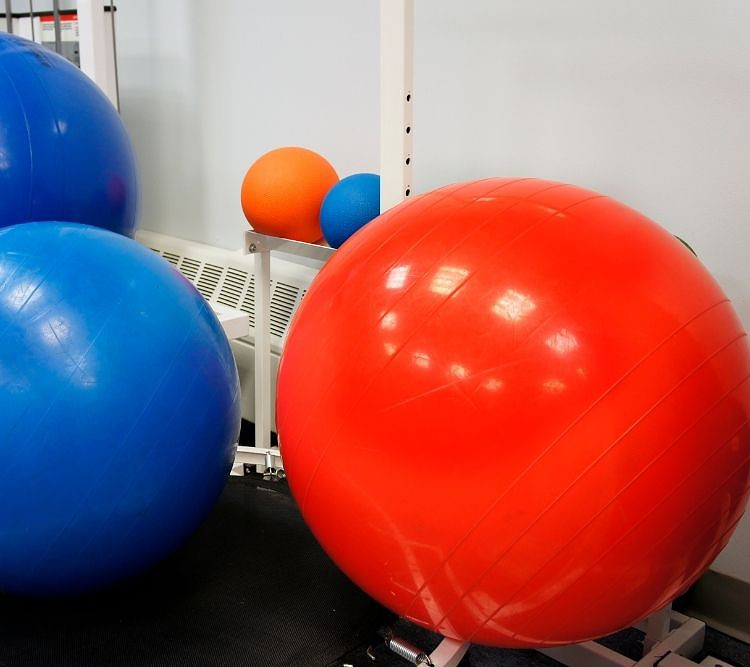Although most exercise balls are the same, they have different names. The different names for exercise balls can be confusing if you’re not familiar with them.

For example, a Swiss ball is another term for a large exercise ball. It was called the Swiss ball after the earliest recorded use of exercise balls in Switzerland.

The standard size of a Swiss ball ranges from 55 to 75 centimeters in diameter. There are smaller-sized Swiss balls  to accommodate those whose height is below 155 centimeters (five feet). The size of these balls is 45 centimeters in diameter.

Pilates ball , also known as an overball, is another term for an exercise ball. It is small or medium in size and used to correct body posture or build muscle and strength.

This ball is nine inches (23 cm) in diameter and has an easy-grip texture making it easier to hold during pilates poses or when using it with other equipment.Some Pilates balls can be as small as eight inches , but this is not the most popular size.

The right pilates size ball would be one that you can easily hold in one hand. The reason for this is some pilates poses require you to hold the ball in one hand.

## Conclusion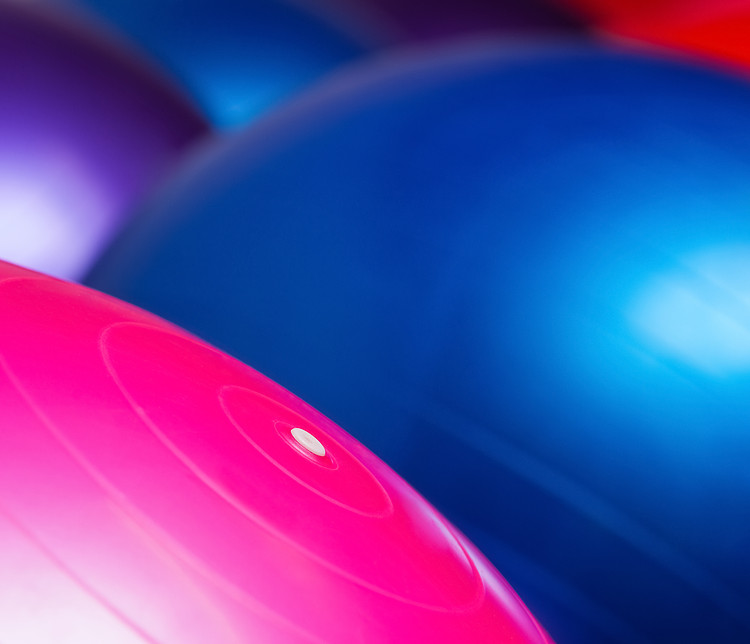There are many types of exercise balls, but the most common one is the large exercise ball you often see in yoga studios and gyms.

A large exercise ball  is measured in diameter using centimeters. To get the size of the ball, you can either use a measuring tape or use a wall and a box to measure the circumference and space the ball uses.

The right exercise ball should allow you to sit upright with your legs at a 90-degree angle.